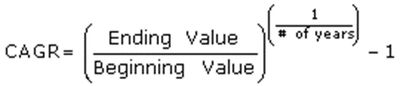#### Ratio Analysis Overview

There are many types of financial ratio analysis but those that will typically be used include:

· Liquidity

· Leverage

· Activity

· Profitability

· Growth

### Tips for Financial Ratio Analysis

Income Statement Tips:

· All line items expressed as a % of revenue should divide by net revenue, not gross

· Break out all operating expenses as much as possible

· Break out interest expense and interest income

· Look at past trends in expenses as a % of revenue

· Find out components of COGS. Is a certain raw material expense about to increase a lot? Do they have a sourcing contract about to roll-off?

Balance Sheet Tips:

· For any ratio based on book value (shareholders’ equity), strip out goodwill. Use tangible book = shareholders’ equity – goodwill.

· For debt ratios, including capitalized leases

· Create a debt table. Is debt about to mature? Will the new rate be higher or lower? Check the maturity schedule.

#### Financial Ratio Analysis - Liquidity

“Liquidity ratios” gauge a company’s ability to meet short-term obligations. Credit analysts at banks use these ratios to monitor their corporate clients, and fixed income managers use them when analyzing companies.

“Current Ratio” =  Current Assets

Current Liabilities

The higher this ratio, the more liquid assets a company has to cover its short-term liabilities.

“Quick Ratio” = Current Assets – Inventory

Current Liabilities

This is a stricter gauge because inventory cannot be “quickly” liquidated to pay bills. A credit analyst would look at this in the context of inventory turns, also known as the “acid test” ratio.

#### Financial Ratio Analysis - Leverage

“Leverage ratios” gauge a company’s risk in the context of its debt levels. Is it generating sufficient EBITDA (D&A is a non-cash expense) to meet its interest payments?

Debt Multiple =   Avg. of Beg/End Year Total Debt

EBITDA

Interest Coverage =  EBITDA + Other Income

Interest

Cost of Debt =  Interest Expense

Avg. of Beg/End Year Total Debt

Note: When calculating any ratio where the numerator is an income statement item and the denominator is a balance sheet item or vice versa, always use an average for the balance sheet item. Income is over some time; the balance sheet is a snapshot in time.

#### Financial Ratio Analysis - Activity

Activity ratios gauge a company’s efficient utilization of its assets.

Inventory Turnover =  COGS

Avg. Inventory

Asset Turnover =   Revenue

Avg. Assets

Days in Receivables = Avg. Accounts Receivable x 360

Revenues

Days in Payables = Avg. Accounts Payable x 360

COGS

Example:

How many times a year does a company turn over its inventory if:

Dec. 31, 2012 inventory = \$100

Dec. 31, 2013 inventory = \$120

COGS in 2013 income statement = \$220

Inventory turnover = \$220/((\$100 + \$120)/2) = 2x

In 2013, they sold twice the inventory they carried on average. So, they sold the entire beginning of year amount, manufactured a second batch, and sold that too.

#### Financial Ratio Analysis - Profitability

“Profitability Ratios” gauge a company’s performance relative to its competitors, and to its historical results.

Gross Margin =  Gross Profit

Revenue

EBIT (operating) Margin =   EBIT

Revenue

EBITDA Margin =  EBITDA

Revenue

Net Margin =  Net Income

Revenue

Remember gross profit = revenue – COGS. EBIT = operating income. EBITDA = EBIT + D&A (from cash flow statement)

#### Financial Ratio Analysis - Growth Stats

Annual growth, such as revenue or EPS growth, year over year is expressed in models as Y/Y.

If this year’s revenue = \$100, last year’s = \$90, the “year over year” growth rate was

\$100/\$90 - 1 = 11.1%

Sequential growth refers to a company’s quarterly results’ growth versus the prior quarter, 90 days prior.

If 3Q was \$20, and 2Q was \$19, the “sequential growth” was \$20/\$19 – 1 = 5.3%

Sequential growth rates are expressed in models as Q-Q and are looked at to gauge if growth is rising or slowing within a year. Quarterly results are also looked at on Y/Y basis, not just Q-Q.#### Financial Ratio Analysis - Growth Stats (CAGR)

Compounded Annual Growth Rate (CAGR, pronounced “kay-ger”) shows the average annual growth rate over some time longer than a year. For counting the number of years, let’s say it’s from 2005-2013, the number of years is 8, not 9. There are 9 years represented, but 8 years elapsed. For instance, from 2005 to 2006, 1 year elapsed, not 2 years.

Example:

2005 revenue = \$10 2013 revenue = \$30

2013 minus 2005 = 8 years

CAGR = \$30/\$10, to the power of (1/8) minus 1  CAGR is 15%